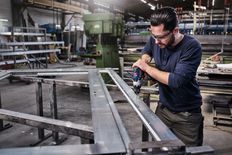Twist Drill Bits

HSS Twist Drill Bit PointTeQ

HSS Twist Drill Bit PointTeQ enables fast drilling in metal due to PointTeQ tip design

New
HSS Twist Drill Bit PointTeQ
HSS Twist Drill Bit PointTeQ enables fast drilling in metal due to PointTeQ tip designProduct highlights

HSS Twist Drill Bit PointTeQ Sets

HSS Twist Drill Bit PointTeQ enables fast drilling in metal due to PointTeQ tip design

• Self-centering tip prevents wandering, making quick and easy drilling starts possible
• Increased drilling rate generates less heat and wear
• PointTeQ tip design remains sharp and ensures continuous durability
Go to product range

Product descriptions

The HSS Twist Drill Bit PointTeQ enables fast drilling in metal due to its PointTeQ tip design. Quick and easy drilling starts are possible as the self-centering tip prevents wandering. Because of its increased drilling rate, less heat and wear are generated. The PointTeQ tip design remains sharp and ensures continuous durability. This bit is suitable for drilling clearance and pilot holes in alloyed and non-alloyed steel, non-ferrous metals, cast steel, cast iron and plastics. It has a cylindrical shank system (shank equal to drill bit diameter) and is intended for use in drill stands and drill drivers.

There is a low risk of breakage, especially for drill bits below 6 mm in diameter as the bit is manufactured to have a highly elastic body. Its black-oxide coated flute provides fast chip removal. The HSS Twist Drill Bit PointTeQ is manufactured to DIN 338. The drill bit is Type N (flute angle) with a tip of 135 degrees.

HSS Twist Drill Bit PointTeQ
HSS Twist Drill Bit PointTeQ enables fast drilling in metal due to PointTeQ tip designSelf-centering tip prevents wandering, making quick and easy drilling starts possibleIncreased drilling rate generates less heat and wearPointTeQ tip design remains sharp and ensures continuous durability

Product range

7 Available product variations
Part number Diameter mm Working length mm Pack quantity
2 608 577 345
2=0 / 3=0 / 4=0 / 5=0 / 6=0 24 / 33 / 43 / 52 / 57 5 Pcs
2 608 577 347
2=0 / 3=0 / 4=0 / 5=0 / 6=0 / 8=0 / 10=0 24 / 33 / 43 / 52 / 57 / 75 / 87 7 Pcs
2 608 577 348
1=0 / 2=0 / 3=0 / 4=0 / 5=0 / 6=0 / 7=0 / 8=0 / 9=0 / 10=0 12 / 24 / 33 / 43 / 52 / 57 / 69 / 75 / 81 / 87 10 Pcs
2 608 577 349
1=5 / 2=0 / 2=5 / 3=0 / 3=2 / 3=5 / 4=0 / 4=5 / 4=8 / 5=0 / 5=5 / 6=0 / 6=5 18 / 24 / 30 / 33 / 36 / 39 / 43 / 47 / 52 / 52 / 57 / 57 / 63 13 Pcs
2 608 577 350
1=0 / 1=5 / 2=0 / 2=0 / 2=5 / 3=0 / 3=0 / 3=5 / 4=0 / 4=0 / 4=5 / 5=0 / 5=5 / 6=0 / 7=0 / 8=0 / 9=0 / 10=0 34 / 40 / 49 / 49 / 57 / 61 / 61 / 70 / 75 / 75 / 80 / 86 / 93 / 93 / 109 / 117 / 125 / 133 18 Pcs
2 608 577 351
1=0 / 1=5 / 2=0 / 2=5 / 3=0 / 3=5 / 4=0 / 4=5 / 5=0 / 5=5 / 6=0 / 6=5 / 7=0 / 7=5 / 8=0 / 8=5 / 9=0 / 9=5 / 10=0 12 / 18 / 24 / 30 / 33 / 39 / 43 / 47 / 52 / 57 / 57 / 63 / 69 / 69 / 75 / 75 / 81 / 81 / 87 19 Pcs
2 608 577 352
1=0 / 1=5 / 2=0 / 2=5 / 3=0 / 3=5 / 4=0 / 4=5 / 5=0 / 5=5 / 6=0 / 6=5 / 7=0 / 7=5 / 8=0 / 8=5 / 9=0 / 9=5 / 10=0 / 10=5 / 11=0 / 11=5 / 12=0 / 12=5 / 13=0 12 / 18 / 24 / 30 / 33 / 39 / 43 / 47 / 52 / 57 / 57 / 63 / 69 / 69 / 75 / 75 / 81 / 81 / 87 / 87 / 94 / 94 / 101 / 101 / 101 25 Pcs

Similar accessories

Check out similar accessories
Service Hotline

Have any questions about our repair and spare parts service?